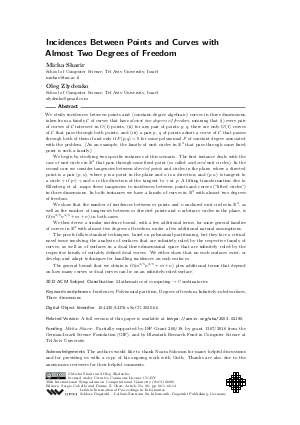Document# Incidences Between Points and Curves with Almost Two Degrees of Freedom

### Authors Micha Sharir, Oleg Zlydenko## File

LIPIcs.SoCG.2020.66.pdf
• Filesize: 492 kB
• 14 pages

## Acknowledgements

The authors would like to thank Noam Solomon for many helpful discussions and for providing us with a copy of his ongoing work with Guth. Thanks are also due to the anonymous reviewers for their helpful comments.

## Cite As

Micha Sharir and Oleg Zlydenko. Incidences Between Points and Curves with Almost Two Degrees of Freedom. In 36th International Symposium on Computational Geometry (SoCG 2020). Leibniz International Proceedings in Informatics (LIPIcs), Volume 164, pp. 66:1-66:14, Schloss Dagstuhl - Leibniz-Zentrum für Informatik (2020)
https://doi.org/10.4230/LIPIcs.SoCG.2020.66

## Abstract

We study incidences between points and (constant-degree algebraic) curves in three dimensions, taken from a family C of curves that have almost two degrees of freedom, meaning that (i) every pair of curves of C intersect in O(1) points, (ii) for any pair of points p, q, there are only O(1) curves of C that pass through both points, and (iii) a pair p, q of points admit a curve of C that passes through both of them if and only if F(p,q)=0 for some polynomial F of constant degree associated with the problem. (As an example, the family of unit circles in ℝ³ that pass through some fixed point is such a family.) We begin by studying two specific instances of this scenario. The first instance deals with the case of unit circles in ℝ³ that pass through some fixed point (so called anchored unit circles). In the second case we consider tangencies between directed points and circles in the plane, where a directed point is a pair (p,u), where p is a point in the plane and u is a direction, and (p,u) is tangent to a circle γ if p ∈ γ and u is the direction of the tangent to γ at p. A lifting transformation due to Ellenberg et al. maps these tangencies to incidences between points and curves ("lifted circles") in three dimensions. In both instances we have a family of curves in ℝ³ with almost two degrees of freedom. We show that the number of incidences between m points and n anchored unit circles in ℝ³, as well as the number of tangencies between m directed points and n arbitrary circles in the plane, is O(m^(3/5)n^(3/5)+m+n) in both cases. We then derive a similar incidence bound, with a few additional terms, for more general families of curves in ℝ³ with almost two degrees of freedom, under a few additional natural assumptions. The proofs follow standard techniques, based on polynomial partitioning, but they face a critical novel issue involving the analysis of surfaces that are infinitely ruled by the respective family of curves, as well as of surfaces in a dual three-dimensional space that are infinitely ruled by the respective family of suitably defined dual curves. We either show that no such surfaces exist, or develop and adapt techniques for handling incidences on such surfaces. The general bound that we obtain is O(m^(3/5)n^(3/5)+m+n) plus additional terms that depend on how many curves or dual curves can lie on an infinitely-ruled surface.

## Subject Classification

##### ACM Subject Classification
• Mathematics of computing → Combinatorics
##### Keywords
• Incidences
• Polynomial partition
• Degrees of freedom
• Infinitely-ruled surfaces
• Three dimensions

## Metrics

• Access Statistics
• Total Accesses (updated on a weekly basis)
0

## References

1. Pankaj K. Agarwal, Eran Nevo, János Pach, Rom Pinchasi, Micha Sharir, and Shakhar Smorodinsky. Lenses in arrangements of pseudo-circles and their applications. J. ACM, 51(2):139-186, 2004.2. David A. Cox, John Little, and Donal O'Shea. Ideals, Varieties, and Algorithms: An Introduction to Computational Algebraic Geometry and Commutative Algebra. Springer-Verlag, Berlin, Heidelberg, 2007.3. Jordan S. Ellenberg, Jozsef Solymosi, and Joshua Zahl. New bounds on curve tangencies and orthogonalities. Discrete Analysis, 22:1-22, 2016.4. Larry Guth. Polynomial partitioning for a set of varieties. Mathematical Proceedings of the Cambridge Philosophical Society, 159(3):459––469, 2015. URL: https://doi.org/10.1017/S0305004115000468.
5. Larry Guth and Nets Hawk Katz. On the Erdőrig os distinct distances problem in the plane. Annals Math., 181:155-–190, 2015.6. Larry Guth and Joshua Zahl. Algebraic curves, rich points, and doubly-ruled surfaces. American Journal of Mathematics, 140:1187-–1229, March 2015.7. Niels Lubbes. Families of circles on surfaces. arXiv preprint, 2013. URL: http://arxiv.org/abs/1302.6710.
8. Adam Marcus and Gábor Tardos. Intersection reverse sequences and geometric applications. Journal of Combinatorial Theory, Series A, 113(4):675-691, 2006. URL: https://doi.org/10.1016/j.jcta.2005.07.002.
9. János Pach and Micha Sharir. On the number of incidences between points and curves. Combinatorics, Probability and Computing, 7:121-127, 1998.10. Micha Sharir, Adam Sheffer, and Joshua Zahl. Improved bounds for incidences between points and circles. Combinatorics, Probability and Computing, 24(3):490––520, 2015.11. Micha Sharir and Noam Solomon. Incidences with curves and surfaces in three dimensions, with applications to distinct and repeated distances. In Proceedings 28th Annual ACM-SIAM Symposium on Discrete Algorithms, SODA '17, pages 2456-2475, 2017.12. Micha Sharir and Oleg Zlydenko. Incidences between points and curves with almost two degrees of freedom. arXiv preprint, 2020. URL: http://arxiv.org/abs/2003.02190.
13. E. Szemerédi and W. T. Trotter. Extremal problems in discrete geometry. Combinatorica, 3(3):381-392, September 1983.X

Feedback for Dagstuhl Publishing